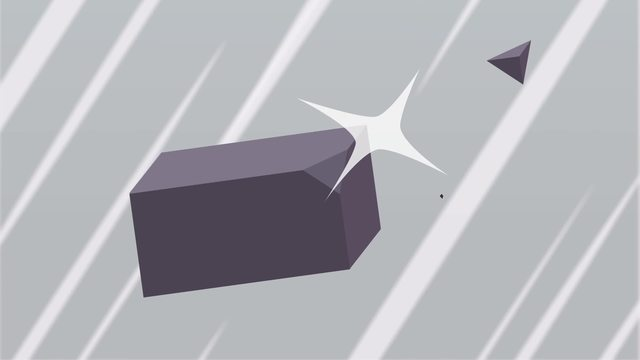# Slicing Right Rectangular Prisms and Pyramids on an AngleRate this video

Ø 4.3 / 4 ratings

The authorChris S.

## Information about the videoSlicing Right Rectangular Prisms and Pyramids on an Angle

After this lesson, you’ll be able to describe and visualize plane sections of slices of right rectangular prisms and pyramids.

The lesson shows you slices of prisms and pyramids not parallel to any face. It then discusses how the number of sides of the plane section equals the number of faces the slice intersects. Finally, you see how the maximum number of sides of a plane section is the number of faces of the 3-d figure.

Learn about slicing prisms and pyramids by helping Yuna become a samurai!

This video includes key concepts, notation, and vocabulary such as quadrilateral (a four-sided polygon); pentagon (a five-sided polygon); hexagon (a six-sided polygon); plane-section (the 2-D figure formed by the intersection of a plane with a 3-D figure); faces (the flat 2-D figures that form a 3-D figure); edges (the segments at the intersection of the faces of a 3-D figure); and vertices (the corner points of the 3-D figure).

Before watching this video, you should already be familiar with slicing prisms parallel to faces and pyramids parallel to the base.

After watching this video, you will be prepared to learn to slice a variety of figures and visualize the resulting plane-sections. You’ll also be prepared to visualize and describe the layers in 3-D figures composed of cubes.

Common Core Standard(s) in focus: 7.G.A.3 A video intended for math students in the 7th grade Recommended for students who are 12 - 13 years old

### TranscriptSlicing Right Rectangular Prisms and Pyramids on an Angle

Yuna has been training for years to master the arts of the samurai warrior. She practices her swordsmanship under the watchful eye of her master, Takeda. But Yuna must take one final test to see if her skills are sharp enough! She must slice right rectangular prisms and pyramids on an angle. Her cut is straight and true, slicing a corner from the box. What was the shape of the box before the slice? It was a right rectangular prism, with 6 rectangular faces. Yuna's slice made a straight cut through the object. That means her blade stayed in a flat PLANE which intersects the prism. The plane isn't parallel or perpendicular to ANY of the prism's faces. What's the shape of the resulting plane-section? Remember, a plane-section is the shape formed by the intersection of a plane with a 3-d figure. The plane-section here is a TRIANGLE. How many FACES did Yuna's slice intersect? Three... which is the same as the number of sides of the plane section. Let's see if that holds true for other slices. Here's a slice that intersects 4 faces. The sides of the plane section are on the faces of the prism, where the plane intersects the face. And there's exactly one side per face, so the number of sides of the plane section is equal to the number of faces intersected. In this case, we get a 4-sided plane section. This actually works for ALL 3-d figures. As long as the plane doesn't intersect a vertex or complete edge, the number of sides of the plane section will be equal to the number of faces intersected by the plane. Going back to our prism, we can create a pentagon with a slicing plane that intersects 5 of the faces, like so. Notice that there are two pairs of parallel lines in this pentagon. Which faces contain THESE two parallel lines? These two faces, which are also parallel! If two parallel faces are both intersected by a slicing plane, the sides they contain will also be parallel. Can we position the slicing plane so that it intersects all 6 faces? Yes! That's a HEXAGON! Is it possible to create a seven-sided plane section by slicing this rectangular prism? No, because that would require us intersecting 7 faces, but this prism only has 6. Runa's next challenge involves a different 3-d figure: a square pyramid. And this time Takeda commands her to create a pentagon plane-section. How many faces will have to be intersected? Our plane will have to intersect all five of the faces to create the five-sided shape. Here's one way that works! But there are also other ways to slice the pyramid and create a pentagon. Where are the VERTICES of the plane-section located? On the EDGES of the pyramid! Noticing that the vertices of the plane section are on the edges of the 3-d figure, and that the sides are on the faces, makes it easier to visualize the plane section. The MAXIMUM number of sides for a plane-section of a square pyramid is 5, because there are only 5 faces to cut but it's possible to create plane-sections that are quadrilaterals and triangles too. All right Yuna, time to prove you are worthy! While Takeda examines the results, let's review what we've learned. When we slice 3-d shapes with planes we create 2-d plane-sections. The sides of the plane-section are always on the FACES of the 3-d figure and the vertices are always on the EDGES. That means the number of sides of the plane-section equals the number of faces intersected. No matter what, the MAXIMUM number of sides possible for a plane-section is the same as the number of faces of the 3-d figure. Yuna passed the test and is now a samuri warrior! The student has become the master... of disaster! Oh Yuna...